Function Repository Resource:

# NFourierTrigSeries

Find a numerical approximation for a trigonometric Fourier series expansion of a function

Contributed by: Wolfram Research
 ResourceFunction["NFourierTrigSeries"][expr,t,n] gives a numerical approximation to the nth-order Fourier trigonometric series expansion of expr in t.

## Details and Options

The numerical approximation to the order n Fourier exponential series expansion of expr is by default defined to be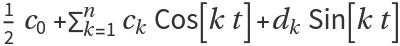.
The coefficient ck is defined to be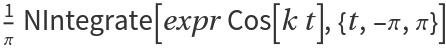and the coefficient dk is defined to be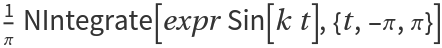.
With the setting FourierParameters{a,b}, the order-n Fourier exponential series expansion computed by ResourceFunction["NFourierTrigSeries"] is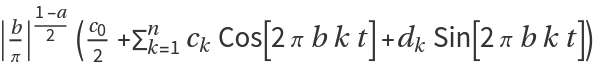. Here, the coefficient ck is defined to be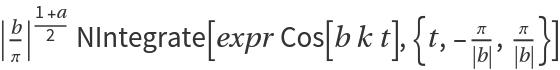and the coefficient dk is defined to be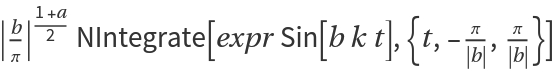.
The parameter b in the setting FourierParameters{a,b} must be numeric.
In addition to the option FourierParameters, ResourceFunction["NFourierTrigSeries"] can also accept the options available to NIntegrate. These options are passed directly to NIntegrate.

## Examples

### Basic Examples (2)

Numerical approximation for a trigonometric Fourier series:

 In:=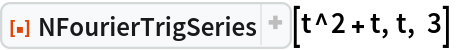Out=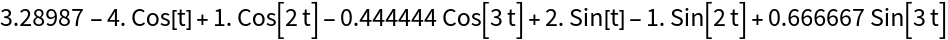In:=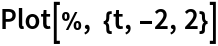Out=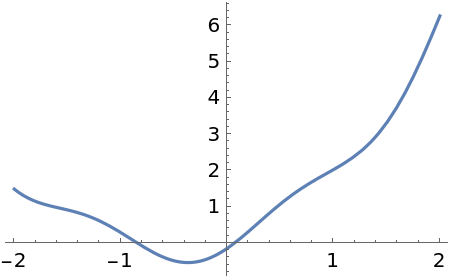Compare with a plot of the original function:

 In:=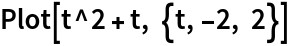Out=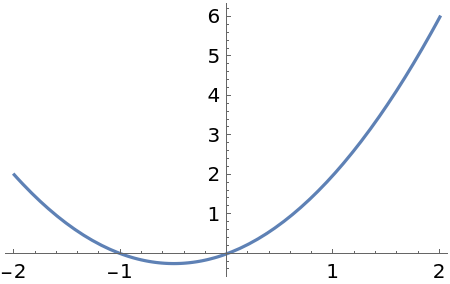## Requirements

Wolfram Language 11.3 (March 2018) or above

## Version History

• 1.0.0 – 12 April 2019